# sim

## Syntax

``simOut = sim(simIn)``
``simOut = sim(simIn,Name,Value)``
``simOut = sim(modelName)``
``````simOut = sim(modelName,Name,Value)``````
``````simOut = sim(modelName,paramStruct)``````
``````simOut = sim(modelName,configSet)``````

## Description

### `Simulink.SimulationInput` Object Syntax

example

````simOut = sim(simIn)` runs one or more simulations of a Simulink® model according to the properties defined on one or more `Simulink.SimulationInput` objects.If `simIn` is a scalar `Simulink.SimulationInput` object, then `simOut` is a scalar `Simulink.SimulationOutput` object.If `simIn` is an array of `Simulink.SimulationInput` objects, then `simOut` is an array of `Simulink.SimulationOutput` objects.You can use a `SimulationInput` object to configure options and inputs for simulations, including: The model to simulateSource variables or files for external input dataBlock parameter values to use for the simulationModel configuration parameter values to use for the simulation When a property of the `SimulationInput` object modifies a model or block parameter value, the value is modified during simulation and reverted at the end of the simulation.When you configure programmatic simulations using `SimulationInput` objects, you can easily transition from using the `sim` function to using other functions, such as `parsim` and `batchsim`.For more information, see Run Simulations Programmatically.```
````simOut = sim(simIn,Name,Value)` simulates a model according to the properties defined on the `Simulink.SimulationInput` object `simIn` with additional options specified using one or more name-value arguments.For a list of name-value arguments supported for the `Simulink.SimulationInput` syntax, see Simulink.SimulationInput Object Syntax.```

### Model Name Syntax

example

````simOut = sim(modelName)` simulates the model specified by `modelName` using the current configuration parameter and block parameter values for the model.If the model has the Single simulation output parameter enabled, `simOut` is a `Simulink.SimulationOutput` object.If the model does not have the Single simulation output parameter enabled, `simOut` is a vector that contains the simulation times. For more information, see Returning multiple output arguments not recommended.```
``````simOut = sim(modelName,Name,Value)``` simulates the model specified by `modelName` with options specified using one or more name-value arguments. For example, you can modify a model configuration parameter value for the simulation by specifying the parameter name and value as a name-value argument.When you modify model configuration parameters by providing inputs to the `sim` function, the changes are applied during simulation and reverted at the end of the simulation.For a list of name-value arguments supported for the model name syntax, see Model Name Syntax.```

example

``````simOut = sim(modelName,paramStruct)``` simulates the model specified by `modelName` using the model configuration parameter values specified by the structure `paramStruct`.```

example

``````simOut = sim(modelName,configSet)``` simulates the model specified by `modelName` using model configuration parameter values in the configuration set `configSet`.```

## Examples

collapse all

You can use a `Simulink.SimulationInput` object to store the configuration for a simulation separate from the model you simulate. The configuration in the `Simulink.SimulationInput` object is applied to the model for the simulation. After simulation, any model settings that were changed revert to the original value.

Open the model `IntegrateSine`. The model uses an Integrator block to integrate the output of a Sine Wave block. The output from the Integrator block is connected to an Outport block.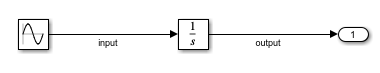```mdl = "IntegrateSine"; open_system(mdl)```

Create a `Simulink.SimulationInput` object to store a simulation configuration for the model `IntegrateSine`.

`simIn = Simulink.SimulationInput(mdl);`

Use the `setModelParameter` function to configure the `SimulationInput` object to use the `ode45` solver and to simulate to a stop time of `20` seconds.

```simIn = setModelParameter(simIn,"Solver","ode45",... "StopTime","20");```

Use the `setBlockParameter` function to configure the `SimulationInput` object to set the Amplitude parameter of the Sine Wave block to `2`.

```blk = strcat(mdl,"/Sine Wave"); simIn = setBlockParameter(simIn,blk,"Amplitude","2");```

Simulate the model using the configuration stored in the `Simulink.SimulationInput` object `simIn`.

`out = sim(simIn);`

The model simulates for `20` seconds, using the `ode45` solver and an amplitude of `2` for the Sine Wave block.When you use an array of `Simulink.SimulationInput` objects to configure a set of simulations, you can use a single call to the `sim` function to run the set of simulations using fast restart. Fast restart saves time in simulation by keeping the model compiled between simulation runs.

Open the model `IntegrateSine`. The model uses an Integrator block to integrate the output of a Sine Wave block. The output from the Integrator block is connected to an Outport block.

```mdl = "IntegrateSine"; open_system(mdl)```Suppose you want to run a set of six simulations that each use a different frequency value for the Sine Wave block. Create a vector that contains the frequency values for the simulations.

`freqs = [0.5 1 1.5 2 2.5 3];`

When you want to tune a block parameter, you can define the value of the parameter using a variable. Then, to tune the block parameter, you change the value of the variable.

Define the variable `freq` to use for the value of the Frequency parameter for the Sine Wave block. For the initial variable value, use the current parameter value.

```blk = mdl + "/Sine Wave"; freq = str2double(get_param(blk,"Frequency"));```

Set the `Frequency` parameter value for the Sine Wave block to `freq`.

`set_param(blk,"Frequency","freq")`

In a `for` loop, create an array of six `Simulink.SimulationInput` objects and use the `setVariable` function to configure each object to use a value from the vector of frequencies.

```for k = length(freqs):-1:1 simIn(k) = Simulink.SimulationInput(mdl); simIn(k) = setVariable(simIn(k),"freq",freqs(k)); end```

Run the simulations defined by the array of `SimulationInput` objects `simIn` using the `sim` function. Enable fast restart using the `UseFastRestart` name-value argument. The `UseFastRestart` name-value argument is supported only when the first input argument is a `Simulink.SimulationInput` object. To use fast restart when the first argument is the name of a model, use the `FastRestart` name-value argument.

`out = sim(simIn,"UseFastRestart","on");`
```[28-Feb-2023 11:45:06] Running simulations... [28-Feb-2023 11:45:11] Completed 1 of 6 simulation runs [28-Feb-2023 11:45:12] Completed 2 of 6 simulation runs [28-Feb-2023 11:45:12] Completed 3 of 6 simulation runs [28-Feb-2023 11:45:12] Completed 4 of 6 simulation runs [28-Feb-2023 11:45:12] Completed 5 of 6 simulation runs [28-Feb-2023 11:45:12] Completed 6 of 6 simulation runs ```

To run the same set of simulations without showing the progress messages, specify the `ShowProgress` name-value argument as `off`.

`out = sim(simIn,"UseFastRestart","on","ShowProgress","off");`

To monitor the progress of the simulations using the Simulation Manager, specify the `ShowSimulationManager` name-value argument as `on`. For more information about the Simulation Manager, see Simulation Manager.

```out = sim(simIn,"UseFastRestart","on",... "ShowProgress","off","ShowSimulationManager","on");```

The simulation output `out` is an array of `Simulink.SimulationOutput` objects that contain the metadata and results for each simulation. The order of `SimulationOutput` objects in the output array matches the order of `SimulationInput` objects in the input array. For example, the `SimulationOutput` object at index `1` contains the results of the simulation configured using the `SimulationInput` object at index `1`.

Access the logged output signal for the results of the first simulation, which used a frequency value of `0.5`.

`youtPt5 = out(1).yout`
```youtPt5 = Simulink.SimulationData.Dataset 'yout' with 1 element Name BlockPath ______ _____________________ 1 [1x1 Signal] output IntegrateSine/Outport - Use braces { } to access, modify, or add elements using index. ```

Open the model `IntegrateSine`. The model uses an Integrator block to integrate the output of a Sine Wave block. The output from the Integrator block is connected to an Outport block.

```mdl = "IntegrateSine"; open_system(mdl);```Simulate the model using the current configuration parameter values.

`out = sim(mdl);`

The simulation runs for `10` seconds, integrating a sine wave with an amplitude of `1`.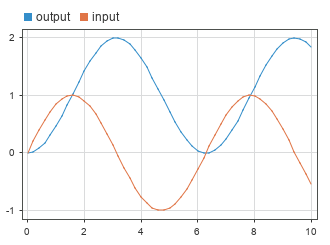You can modify model configuration parameter values and block parameter values in the model using the `set_param` function.

Configure the model to use the `ode45` solver and a stop time of `20` seconds.

`set_param(mdl,"Solver","ode45","StopTime","20")`

Set the Amplitude parameter of the Sine Wave block to `2`.

```blk = strcat(mdl,"/Sine Wave"); set_param(blk,"Amplitude","2")```

When you modify a configuration parameter value or block parameter value using the `set_param` function, the change applies to the block diagram and dirties the model file. When you call the `sim` function again, the simulation uses the new parameter values, which are part of the current model configuration, even if you do not save the model.

`out2 = sim(mdl);`

The simulation runs for `20` seconds, integrating a sine wave with an amplitude of `2`.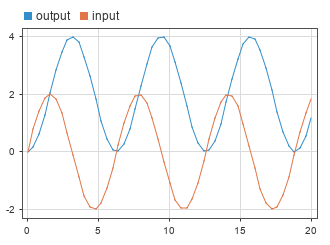You can configure a simulation of a model to use different values for model configuration parameter values by specifying the configuration parameters for the simulation as name-value arguments for the `sim` function. The parameter values you specified are applied for the simulation and reverted when the simulation completes.

You can specify only model configuration parameter values and not block parameter values as name-value arguments for the `sim` function. To specify model configuration parameter values, block parameter values, and variable values for a simulation in a single input, use a `Simulink.SimulationInput` object instead.

Open the model `IntegrateSine`. The model uses an Integrator block to integrate the output of a Sine Wave block. The output from the Integrator block is connected to an Outport block.

```mdl = "IntegrateSine"; open_system(mdl);```Suppose you want to simulate the model using the solver `ode45` and a stop time of `20` seconds. Specify the `Solver` and `StopTime` values for the simulation as name-value arguments for the `sim` function.

`out = sim(mdl,"Solver","ode45","StopTime","20");`

The model simulates through a simulation time of `20` seconds using the solver `ode45`.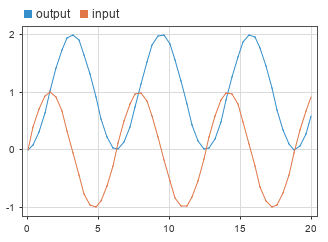You can use a structure of model configuration parameter names and values to configure a simulation of a model. The configuration parameter values in the structure are applied to the model for the simulation. After simulation, any model settings that changed are reverted to the original value.

The structure input can specify only model configuration parameter values and cannot specify different block parameter or variable values to use in the simulation. To specify model configuration parameter values, block parameter values, and variable values for a simulation in a single input, use a `Simulink.SimulationInput` object instead.

Open the model `IntegrateSine`. The model uses an Integrator block to integrate the output of a Sine Wave block. The output from the Integrator block is connected to an Outport block.

```mdl = "IntegrateSine"; open_system(mdl)```Create the structure `SimConfig` that configures a simulation to use the `ode45` solver and a stop time of `20` seconds. The structure contains a field for each configuration parameter to modify in the simulation. The name of each field matches the programmatic name for each parameter. The value of each field specifies the value to use for that parameter in the simulation.

```simConfig.Solver = "ode45"; simConfig.StopTime = "20";```

Simulate the model using the model configuration parameter values from the structure.

`out = sim(mdl,simConfig);`

The model simulates through a simulation time of `20` seconds using the `ode45` solver.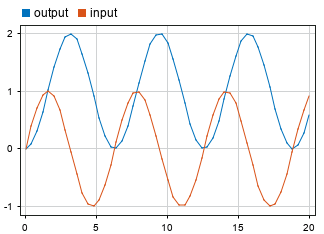A `Simulink.ConfigSet` object stores a set of model configuration parameter values. You can specify a `Simulink.ConfigSet` object as an input to the `sim` function. The configuration set from the object is applied to the model for the simulation. After simulation, the original configuration set is restored in the model.

A `Simulink.Configset` object stores only model configuration parameter values. To specify model configuration parameter values, block parameter values, and variable values for a simulation in a single input, use a `Simulink.SimulationInput` object instead.

Open the model `IntegrateSine`. The model uses an Integrator block to integrate the output of a Sine Wave block. The output from the Integrator block is connected to an Outport block.

```mdl = "IntegrateSine"; open_system(mdl)```Use the `getActiveConfigSet` function to get a `Simulink.ConfigSet` object for the current model configuration.

`mdlConfig = getActiveConfigSet(mdl);`

Use the `copy` function to create a copy of the `Simulink.ConfigSet` object to modify.

`simConfig = copy(mdlConfig);`

Modify the `Simulink.ConfigSet` object `simConfig` to use the solver `ode45` and a stop time of `20` seconds.

`set_param(simConfig,"Solver","ode45","StopTime","20");`

Simulate the model using the configuration parameters in the `Simulink.Configset` object `simConfig`.

`out = sim(mdl,simConfig);`

The model simulates through a simulation time of `20` seconds using the `ode45` solver.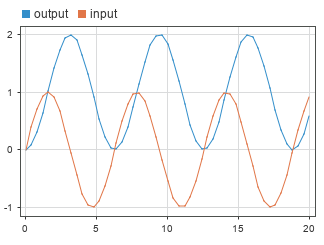## Input Arguments

collapse all

Simulation configuration, specified as a `Simulink.SimulationInput` object. The properties of the `SimulationInput` object specify options and parameter values to use in the simulation, including:

• The model to simulate

• Source variables or files for external input data

• Block parameter values to use for the simulation

• Model configuration parameter values to use for the simulation

The values defined in the properties of the `SimulationInput` object are applied to the model for the simulation and reverted at the end of simulation.

Model to simulate, specified as a string or a character vector.

Example: `simOut = sim("vdp")` simulates the model named `vdp` using the parameter values currently configured in the model.

Data Types: `char` | `string`

Model configuration to simulate, specified as a structure. The fields of the structure are the names of model configuration parameters. The value for each field indicates the parameter value to use in simulation. For example, to simulate a model from a start time of `5` to a stop time of `10`, create this structure:

```paramStruct.StartTime = "5"; paramStruct.StopTime = "10";```

Data Types: `struct`

Model configuration to simulate, specified as a `Simulink.ConfigSet` object.

### Name-Value Arguments

Specify optional pairs of arguments as `Name1=Value1,...,NameN=ValueN`, where `Name` is the argument name and `Value` is the corresponding value. Name-value arguments must appear after other arguments, but the order of the pairs does not matter.

Before R2021a, use commas to separate each name and value, and enclose `Name` in quotes.

Example: `simOut = sim(simIn,"UseFastRestart","on")` runs a set of simulations configured using an array of `Simulink.SimulationInput` objects with fast restart enabled.

Example: `simOut = sim(modelName,"Solver","ode15s","StopTime","30")` configures a simulation of the model specified by `modelName` to use the ode15s solver with a stop time of `30`.

The `sim` function supports different name-value arguments depending on whether you specify the first input as a `Simulink.SimulationInput` object or as the name of the model to simulate. In addition to the arguments listed on this page, you can specify values for model configuration parameters using inputs to the `sim` function.

• When the first input argument is a `Simulink.SimulationInput` object, configure model parameter values for the simulation on the input object using the `setModelParameter` function.

• When the first input argument is the model name, specify any model configuration parameter as a name-value argument.

`Simulink.SimulationInput` Object Syntax

collapse all

Option to enable fast restart, specified as `'off'` or `'on'`. Fast restart reduces the time required to run a set of simulations by skipping the compilation and termination phases when appropriate. Consider using fast restart when you run multiple simulations of the same model.

This argument is supported only when you specify the first input argument for the `sim` function as a `Simulink.SimulationInput` object.

Example: `sim(simIn,"UseFastRestart","on")`

Data Types: `char` | `string`

Option to stop process that started simulation when error occurs, specified as `'off'` or `'on'`.

• `'off'` — When an error occurs in a simulation, that simulation stops and the process that started the simulation continues. For example, when you run a set of simulations using an array of `Simulink.SimulationInput` objects, if the first simulation encounters an error, that simulation stops and subsequent simulations still run.

• `'on'` — When an error occurs in a simulation, that simulation and the process that started the simulation both stop. For example, when you run a set of simulations using an array of `Simulink.SimulationInput` objects, if the first simulation encounters an error, that simulation stops and subsequent simulations do not run.

This argument is supported only when you specify the first input argument for the `sim` function as a `Simulink.SimulationInput` object.

Example: `sim(simIn,"StopOnError","on")`

#### Tips

• When you specify the name of the model as the first input argument for the `sim` function, configure this behavior using the `CaptureErrors` name-value argument.

• When an error does not stop the process that started a simulation, error message information is captured in the `Simulink.SimulationOutput` object and the `Simulink.SimulationMetadata` object.

• To view the message, use the `ErrorMessage` property of the `SimulationOutput` object.

• For more information about the error, use the `ExecutionInfo` property of the `Simulink.SimulationMetadata` object. The `ErrorDiagnostic` field includes information about the error, including the simulation phase where the error occurred.

Data Types: `char` | `string`

Option to indicate simulation progress, specified as `'off'` or `'on'`.

• `'off'` — Simulations run without displaying progress messages.

• `'on'` — Progress updates are displayed as simulations progress.

The default value for this parameter depends on the size of the first input argument:

• When the first input argument is a scalar `Simulink.SimulationInput` object, the default value is `'off'`.

• When the first input argument is an array of `Simulink.SimulationInput` objects, the default value is `'on'`.

This argument is supported only when you specify the first input argument for the `sim` function as a `Simulink.SimulationInput` object.

Example: `sim(simIn,"ShowProgress","on")`

Option to open Simulation Manager, specified as `'off'` or `'on'`. Use the Simulation Manager to monitor the progress of simulations you run. Consider using the Simulation Manager when you run multiple simulations using an array of `Simulink.SimulationInput` objects.

This argument is supported only when you specify the first input argument for the `sim` function as a `Simulink.SimulationInput` object.

Example: `sim(simIn,"ShowSimulationManager","on")`

Model Name Syntax

collapse all

Option to continue process that started simulation if error occurs, specified as `'off'` or `'on'`. By default, when you run a simulation using the `sim` function and specify the name of the model as the first input:

• Errors are reported in the MATLAB® Command Window.

• Both the simulation and the process that invoked the simulation stop when the error occurs.

• The error message is not captured in the `Simulink.SimulationOutput` object or `Simulink.SimulationMetadata` object.

When you specify `CaptureErrors` as `'on'`, errors are reported only in the simulation outputs. The execution of the simulation with the error stops, but if the simulation was invoked by another process, that process continues. For example, when you run multiple simulations in a loop, if you specify `CaptureErrors` as `'on'`, subsequent simulations continue to run following a simulation with an error.

This argument is supported only when you specify the first input argument for the `sim` function as the name of the model to simulate.

Example: `sim("myModel","CaptureErrors","on")`

#### Tips

• This option is not supported for software-in-the-loop (SIL) and processor-in-the-loop (PIL) simulations.

• When you specify one or more `Simulink.SimulationInput` objects as input to the `sim` function, configure this behavior using the `StopOnError` name-value argument.

• When you specify `CaptureErrors` as `'on'`, error message information is captured in the `Simulink.SimulationOutput` object and the `Simulink.SimulationMetadata` object.

• To view the message, use the `ErrorMessage` property of the `SimulationOutput` object.

• For more information about the error, use the `ExecutionInfo` property of the `Simulink.SimulationMetadata` object. The `ErrorDiagnostic` field includes information about the error, including the simulation phase where the error occurred.

Data Types: `char` | `string`

Option to start simulation in debug mode, specified as `'off'` or `'on'`.

This argument is supported only when you specify the first input argument for the `sim` function as the name of the model to simulate.

Example: `sim("modelName","Debug","on")`

Data Types: `char` | `string`

Option to disable rebuilding rapid accelerator target, specified as `'on'` or `'off'`. When you specify this argument as `'on'`, changes that require rebuilding the rapid accelerator target are ignored. When you use this option, modify only options that do not require rebuilding the rapid accelerator target.

This argument is supported only when you specify the first input argument for the `sim` function as the name of the model to simulate.

Example: `sim("modelName","RapidAcceleratorUpToDateCheck","off")`

#### Tips

To specify this option for a simulation configured using a `Simulink.SimulationInput` object, use the `setModelParameter` function.

```simIn = Simulink.SimulationInput("myModel"); simIn = setModelParameter(simIn,"RapidAcceleratorUpToDateCheck","off");```

Data Types: `char` | `string`

Maximum simulation run time, specified as a positive scalar. Specify the time, in seconds, to allow the simulation to run. If the simulation runs for longer than the value you specify, the software issues a warning and stops the simulation. For example, if you specify `TimeOut` as `30`, the software stops the simulation and issues a warning if computing simulation results takes more than 30 seconds.

The `TimeOut` parameter specifies a limit on the amount of clock time for a simulation to run. To specify the maximum time value to simulate, use the Stop time parameter.

This argument is supported only when you specify the first input argument for the `sim` function as the name of the model to simulate.

Example: `sim("modelName","TimeOut",60)` configures a simulation to run for a maximum duration of 60 seconds.

#### Tips

• Consider specifying a timeout when you run multiple variable-step simulations in serial. If simulation conditions constrain the step size so that the solver starts taking many very small time steps, the simulation can time out so that subsequent simulations can run.

• To specify this option for a simulation configured using a `Simulink.SimulationInput` object, use the `setModelParameter` function.

```simIn = Simulink.SimulationInput("modelName"); simIn = setModelParameter(simIn,"TimeOut",60);```

Option to display summary of parameters before simulation, specified as `'siminfo'`.

This argument is supported only when you specify the first input argument for the `sim` function as the name of the model to simulate.

Example: `sim("modelName","Trace","siminfo")`

Data Types: `char` | `string`

## Output Arguments

collapse all

Simulation outputs, returned as a `Simulink.SimulationOutput` object, an array of `Simulink.SimulationOutput` objects, or a vector. The `Simulink.SimulationOutput` object contains all data logged from simulation as well as metadata about the simulation, including timing information and diagnostics.

When you specify only the model name as an input argument and the model you simulate has the Single simulation output parameter disabled, the output from the `sim` function is a vector of simulation times. For the `sim` function to return results in a consistent format for any syntax, save the model with the Single simulation output parameter enabled.

## Tips

• To ensure the `sim` function returns results in the same format regardless of which input arguments you specify, save your model with the Single simulation output parameter enabled. With this option enabled, simulation results are returned as a `Simulink.SimulationOutput` that contains all logged data as well as simulation metadata, including timing information and diagnostics. Analyzing results from multiple simulations is easier when all simulation data and metadata is stored in a single object.

• To get a list of model configuration parameters, use the `getActiveConfigSet` function and the `get_param` function. For example, to see the configuration parameters for the model `vdp`, enter these commands in the MATLAB Command Window.

```configSet = getActiveConfigSet("vdp"); configSetNames = get_param(configSet,"ObjectParameters")```

The return from the `get_param` function lists the model configuration parameters such as `StopTime`, `SaveTime`, `SaveState`, `SaveOutput`, and `SignalLogging`.

• When you simulate a model hierarchy, model configuration parameters you specify as input arguments to the `sim` function apply to the top model.

• When you run a simulation using the `sim` function, the simulation runs until an error occurs or the simulation reaches the specified stop time. To programmatically run an interactive simulation that you can pause and continue programmatically, use the `set_param` function with the `SimulationCommand` input argument. For more information, see Run Simulations Programmatically.

• When you simulate a model with infinite stop time, stop the simulation from the MATLAB Command Window by pressing Ctrl+C. The simulation stops, and simulation results are not saved in the MATLAB workspace.

• Configure logging for time, states, and outputs using the Configuration Parameters dialog box. On the Modeling tab, under Setup, click Model Settings. Then, in the Configuration Parameters dialog box, select Data Import/Export.

• To log signals throughout a model, use signal logging or logging blocks such as the To Workspace block or the Record, XY Graph block. For more information about signal logging, see Save Signal Data Using Signal Logging.

## Version History

Introduced before R2006a

expand all